# Process Capability

Process Capability is a quality index that specifies the ability of your manufacturing process to produce acceptable parts. It is a fundamental aspect of Statistical Process Control in that it represents a metric of the variation of the process in relationship to the tolerance range and includes deviation of process mean to nominal target.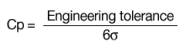Given: Engineering Specifications = 60 ± 5; USL = 65, LSL = 55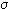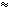s=2.3232
Since Cp depends on the unknown value of, we will use an estimate of(which is s) to estimate Cp.

Step 1: Calculate the engineering Tolerance.
Engineering tolerance is 65 – 55 = 10

Step 2: Estimate capability.
Process capability = 66s = 6 x 2.3232 = 13.9392

Step 3: Estimate Cp.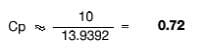Step 4: Estimate Cpk.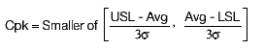Given: Engineering specifications = 60 ± 5; USL = 65, LSL = 55.
Avg = 60.15s=2.3232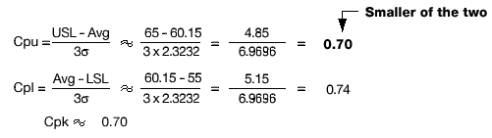Calculating Cpk for Specific Cases:

Case 1: Upper and lower specifications are provided and engineering nominal (or target) is centered between the specification limits.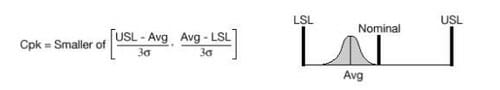Case 2: A lower physical bound is used as the lower specification limit, or no lower specification exists. It is assumed that smaller feature measurements are always superior to larger values.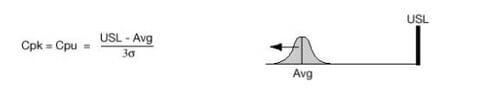Case 3: An upper physical bound is used as the upper specification limit, or no upper specification exists. It is assumed that larger feature measurements are always superior to smaller values.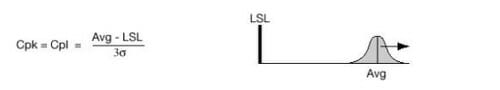Case 4: Upper, lower, and engineering nominal (or target) specifications are given, but nominal is closer to the lower specification than the upper specification.

Cpk is maximized when the process average equals the nominal specification. Cpk is positive when the process average lies between the upper and lower specification limits, and is 0.0 when the process average equals either LSL or USL. When nominal is not centered between the upper and lower specification limit, a higher Cp is required to meet a Cpk of 1.33 than if the nominal had been centered.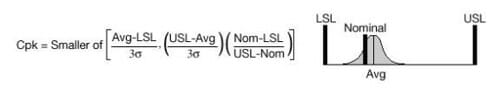Case 5: Upper, lower, and engineering nominal (or target) specifications are given, but nominal is closer to the upper specification than the lower specification. Cpk is maximized when the process average equals the nominal specification. Cpk is positive when the process average lies between the upper and lower specification limits, and is 0.0 when the process average equals either LSL or USL. When nominal is not centered between the upper and lower specification limit, a higher Cp is required to meet a Cpk of 1.33 than if the nominal had been centered.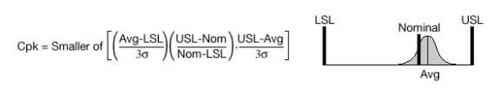Case 6: Upper, lower, and engineering nominal (or target) specifications are given, but the nominal is equal to the lower specification limit and there are no physical bounds limiting measurements from going below nominal.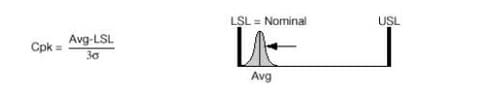For this case and the following case only, a large Cpk is not desirable. The optimal Cpk is 1.33, and Cp should be maximized instead of Cpk.

Case 7: Upper, lower, and engineering nominal (or target) specifications are given, but the nominal is equal to the upper specification limit and there are no physical bounds limiting measurements from going above nominal.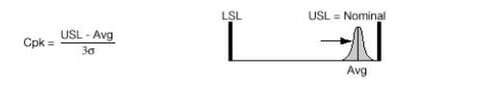For this case and the preceding case only, a large Cpk is not desirable. The optimal Cpk is 1.33, and Cp should be maximized instead of Cpk.

When no nominal is given, a manufacturing target should be established — generally halfway between the upper and lower specifications. In such instances, use case 1, 4, 5, 6, or 7, as appropriate.